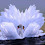#### WhatsApp trending coin puzzle with full answer

7

Explanation
Equation 1: 10 + 10 + 10 = 30
Equation 2: 5 + 5 + 2 = 12
Equation 3: 10 + 1 + 5 = 16
Equation 4: (5 + 4) - (10 + 2) + (1 + 10 + 1) = 7

1.How it is possible

2.(5+2)-(2+10)+(1+10+1) = 7

3.This comment has been removed by the author.